## Gaussian Distribution Linear Combination of Variates

Ifis Normally Distributed with Meanand Variance, then a linear function of,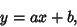(1)

is also Normally Distributed. The new distribution has Meanand Variance, as can be derived using the Moment-Generating Function(2)

which is of the standard form with(3)(4)

For a weighted sum of independent variables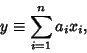(5)

the expectation is given by(6)

Setting this equal to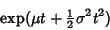(7)

gives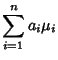(8)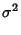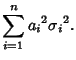(9)

Therefore, the Mean and Variance of the weighted sums ofRandom Variables are their weighted sums.

Ifare Independent and Normally Distributed with Mean 0 and Variance, define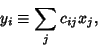(10)

where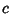obeys the Orthogonality Condition(11)

with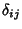the Kronecker Delta. Thenare also independent and normally distributed with Mean 0 and Variance.